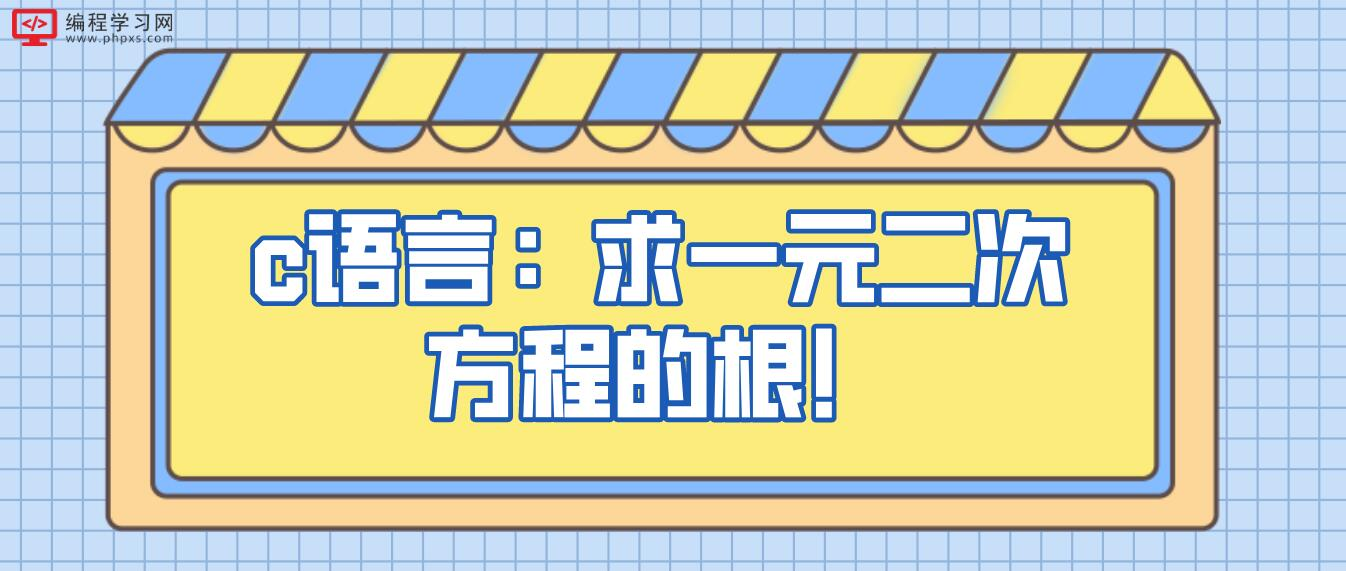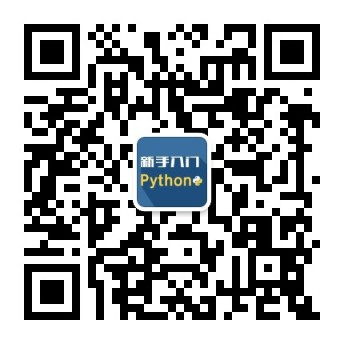2022
10-29

# c语言：求一元二次方程的根！#include <stdio.h>
#include <math.h>
double a = 0, b = 0, c = 0, p, q;
int main(void)
{
printf("请分别输入a,b,c三个值并用空格隔开，按回车开始运算：\n");
scanf("%lf %lf %lf", &a, &b, &c);
if(a == 0 )
printf("此一元一次方程的解为x= %lf\n", -c/b);
else if (b*b-4*a*c<0)
printf("此方程无解\n");
else
{
p = -b/2*a;
q = (sqrt(b*b-4*a*c)/2*a);
printf("此一元二次方程的解为x1= %lf,x2= %lf\n该抛物线的对称轴为：= %lf\n最大/最小值为: = %lf", p+q,p-q, -b/2*a,(4*a*c-b*b)/4*a);

system("pause");
return 0;

1.首先，要去网上搜集几个你中意的ico图片文件来当他的图标。
2.在DEV C++左侧项目管理功能栏中找到项目，单击右键，弹出一个选择框，选择项目属性。